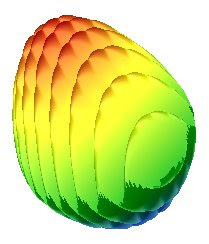### Mathematical egg

Equation: $1.2 + \left ( \sqrt{\left (1 - \sqrt{x^2+y^2} \right )^2} + 1 - x^2 - y^2 \right ) \cdot \left ( \sin \left ( 10 \left ( \frac{3x + y}{5} + 7 \right ) \right ) + \frac{1}{4} \right )$ Instruction for Google search box:
1.2+(sqrt(1-(sqrt(x^2+y^2))^2) + 1 - x^2-y^2) * (sin (10 * (x*3+y/5+7))+1/4)
Result: